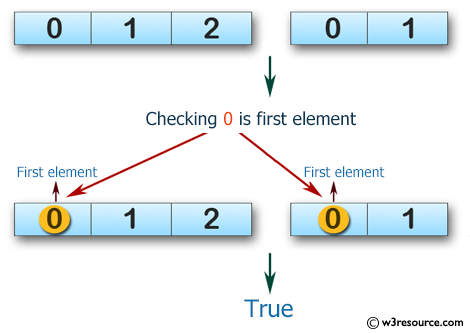﻿ Swift Array Programming Exercise: Check if two given arrays of integers have 0 as their first element - w3resource# Swift Array Programming Exercises: Check if two given arrays of integers have 0 as their first element

## Swift Array Programming: Exercise-15 with Solution

Write a Swift program to check if two given arrays of integers have 0 as their first element.

Pictorial Presentation:Sample Solution:

Swift Code:

``````func start1(_ a: [Int], _ b: [Int]) -> Bool {
var ctr = 0

if a.first == 0
{
ctr += 1
}

if b.first == 0
{
ctr += 1
}

if  ctr == 2
{
return true
}
else
{
return false
}

}

print(start1([0, 1, 2], [0, 1]))
print(start1([0, 1, 2], [1, 2]))
print(start1([0, 1], ))
```
```

Sample Output:

```true
false
true
```

Swift Programming Code Editor:

Improve this sample solution and post your code through Disqus

What is the difficulty level of this exercise?

﻿